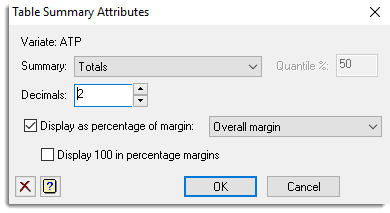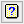1. Home
2. Multiple Summary Tables Attributes

# Multiple Summary Tables Attributes

This dialog lets you change the summary options for the selected items in the Multiple Summary Tables menu.## Variate

If just a single item is selected, its name is displayed here, otherwise it will display Multiple variates.

## Summary

Select the statistics that you want to produce.

 Means Mean of the sample for each group Standard deviations Standard deviation of the sample for each group Medians Median value that divides each grouped sample into two equally sized sets Totals Totals of values within groups Number of observations Number of observations within each group Minima Minimum value within each group Maxima Maximum value within each group Variances Variance of the samples for each group Quantiles A specified quantile of the samples for each group. Specify the quantiles required within the Quantile % field. Skewness Skewness of the samples for each group Kurtosis Kurtosis of the samples for each group Standard error of mean Standard error of mean of the samples for each group Standard error of skewness Standard error of skewness of the samples for each group Standard error of kurtosis Standard error of kurtosis of the samples for each group Counts Counts of the samples in each group (including missing values)

Note as Counts does not have a variate associated with it, you cannot change an item from Counts to another summary statistic or vice versa.

## Quantile %

Specifies a percentage point for which the quantile will be calculated.

## Decimals

Number of decimal places for numbers. If omitted, a default is determined which outputs the mean absolute value to 4 significant figures.

## Display as percentage of margin

This allows the body of the table to be expressed as percentages of the corresponding values of the selected margin. The margin to be used as the denominator can be chosen by selecting the required set of factors from the dropdown list following the check box.

## Display 100 in percentage margins

When a summary item has Display as percentage of margin selected and Set margins option is also selected, then this controls what numbers are put in the margins of the percentage tables. If this is selected, then 100 is put in the margins, otherwise the summary statistic used to divide the cells to create the percentages is put in the margins.

## Action IconsClear Clear all fields and list boxes.Help Open the Help topic for this dialog.